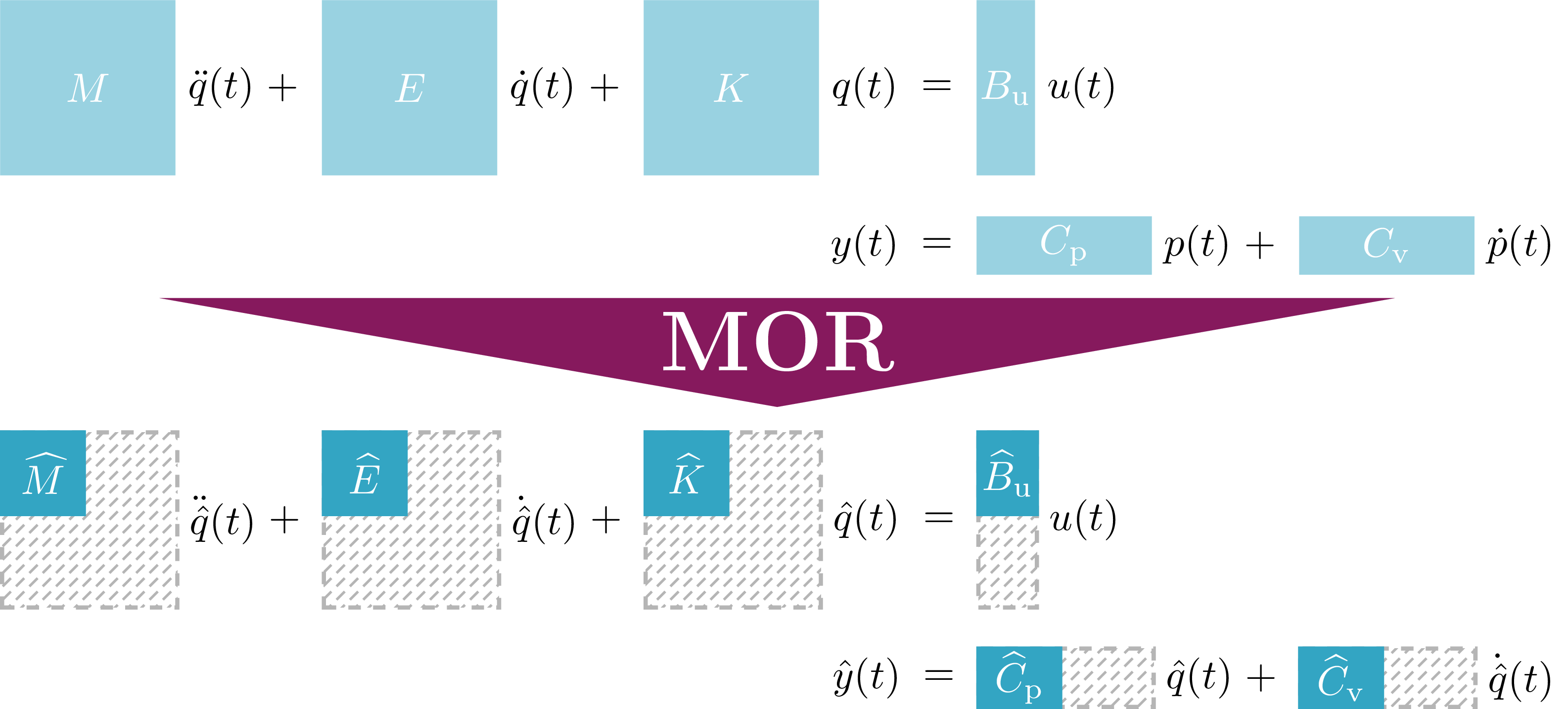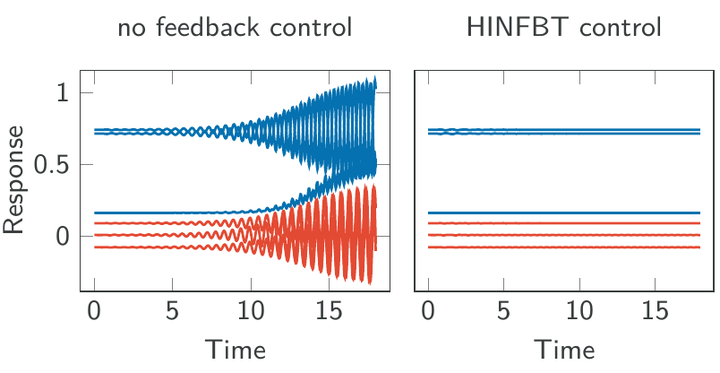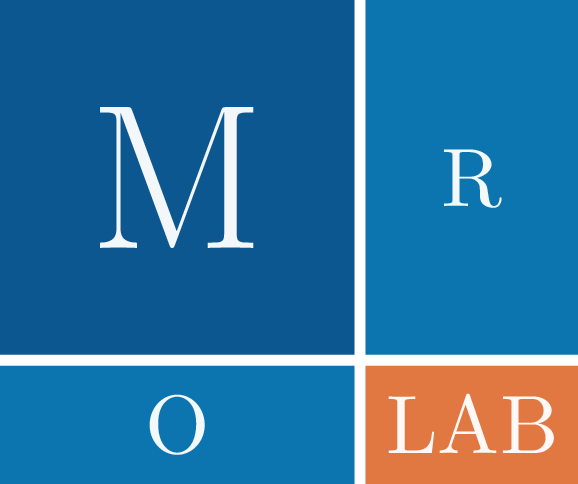# Research Projects

## Research Interests

• Model order reduction: approximation of dynamical systems, structured interpolation, data-driven reduced-order modeling
• Numerical linear algebra: matrix equations, robust controller design, differential-algebraic equations
• Scientific computing: mathematical software, efficient algorithm design, numerical experiments

## Structure-Preserving Model Reduction for Mechanical SystemsFor the construction of complex mechanical structures such as bridges, buildings, or vehicles, the use of computer models is nowadays indispensable. Such computer models are typically used to simulate and, if necessary, to optimize the oscillation behavior. For instance, such an optimization is useful to suppress and damp undesired oscillations. Taking into account the input-output structure of the system, modeling of such mechanical structures typically leads to dynamical systems described by second-order ordinary differential equations. Often the system dimension (i. e., the number of masses) is very large. The aim of model reduction is now to approximate the original system by a much smaller one to save a huge amount of computational resources during evaluation of the model. In this project we want to develop new model reduction techniques for second-order mechanical systems that also preserve certain system properties like dissipativity.

## Robust Flow Control via HINFBTFor the efficient stabilization of flows, controllers of small orders are needed, which also bridge the gap between approximation and model, or model and reality. While there are established construction formulas for robust stabilizing controllers, those usually lead to controllers described by systems of differential equations of the same size as the original system, which makes real time control of the system impossible. In this project, we aim for the construction of such low-order robust stabilizing controllers by employing LQG- and H-Infinity-based model reduction methods and controller theory.

## MORLAB – Model Order Reduction LABoratoryMORLAB, the Model Order Reduction LABoratory toolbox, is a collection of MATLAB and Octave routines for model order reduction of dense linear time-invariant continuous-time systems. The toolbox contains model reduction methods for standard, descriptor and second-order systems based on the solution of matrix equations. Therefore, also spectral projection based methods for the solution of the corresponding matrix equations are included. See the project website for more information.